# 一文学会递归解题

danielmou

0收藏

### 前言

1. 什么是递归？
2. 递归算法通用解决思路
3. 实战演练（从初级到高阶）

``````public int factorial(int n){
if (n < =1) {
return1;
}
return n * factorial(n - 1)
}``````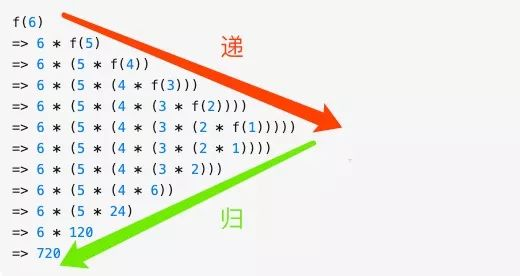1. 一个问题可以分解成具有相同解决思路的子问题，子子问题，换句话说这些问题都能调用同一个函数
2. 经过层层分解的子问题最后一定是有一个不能再分解的固定值的（即终止条件）,如果没有的话,就无穷无尽地分解子问题了，问题显然是无解的。

1. 先定义一个函数，明确这个函数的功能，由于递归的特点是问题和子问题都会调用函数自身，所以这个函数的功能一旦确定了， 之后只要找寻问题与子问题的递归关系即可
2. 接下来寻找问题与子问题间的关系（即递推公式），这样由于问题与子问题具有相同解决思路，只要子问题调用步骤 1 定义好的函数，问题即可解决。所谓的关系最好能用一个公式表示出来，比如f(n) = n * f(n-)这样，如果暂时无法得出明确的公式，用伪代码表示也是可以的, 发现递推关系后，要寻找最终不可再分解的子问题的解，即（临界条件），确保子问题不会无限分解下去。由于第一步我们已经定义了这个函数的功能，所以当问题拆分成子问题时，子问题可以调用步骤 1 定义的函数，符合递归的条件（函数里调用自身）
3. 将第二步的递推公式用代码表示出来补充到步骤 1 定义的函数中
4. 最后也是很关键的一步，根据问题与子问题的关系，推导出时间复杂度,如果发现递归时间复杂度不可接受，则需转换思路对其进行改造，看下是否有更靠谱的解法

### 实战演练（从初级到高阶）

#### 热身赛

1. 定义这个函数，明确这个函数的功能，我们知道这个函数的功能是求 n 的阶乘, 之后求 n-1, n-2 的阶乘就可以调用此函数了

``````/**
* 求 n 的阶乘
*/
public int factorial(int n){
}``````

2.寻找问题与子问题的关系 阶乘的关系比较简单， 我们以 f(n) 来表示 n 的阶乘， 显然 f(n) = n * f(n - 1),  同时临界条件是 f(1) = 1,即

3.将第二步的递推公式用代码表示出来补充到步骤 1 定义的函数中

``````/**
* 求 n 的阶乘
*/
public int factorial(int n){
// 第二步的临界条件
if (n < =1) {
return1;
}

// 第二步的递推公式
return n * factorial(n-1)
}``````

4.求时间复杂度 由于  f(n) = n * f(n-1) = n * (n-1) * .... * f(1),总共作了 n 次乘法，所以时间复杂度为 n。

#### 入门题

`​一只青蛙可以一次跳 1 级台阶或一次跳 2 级台阶,例如:​`​​`​跳上第 1 级台阶只有一种跳法：直接跳 1 级即可。跳上第 2 级台阶​`​​`​有两种跳法：每次跳 1 级，跳两次；或者一次跳 2 级。​`​​`​问要跳上第 n 级台阶有多少种跳法？​`

1.定义一个函数，这个函数代表了跳上 n 级台阶的跳法

``````/**
* 跳 n 极台阶的跳法
*/
public int f(int n){
}``````

2.寻找问题与子问题之前的关系 这两者之前的关系初看确实看不出什么头绪，但仔细看题目，一只青蛙只能跳一步或两步台阶，自上而下地思考，也就是说如果要跳到 n 级台阶只能从 从 n-1 或 n-2 级跳， 所以问题就转化为跳上 n-1 和 n-2 级台阶的跳法了，如果 f(n) 代表跳到 n 级台阶的跳法，那么从以上分析可得 f(n) = f(n-1) + f(n-2),显然这就是我们要找的问题与子问题的关系,而显然当 n = 1, n = 2， 即跳一二级台阶是问题的最终解，于是递推公式系为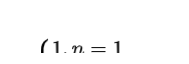3.将第二步的递推公式用代码表示出来补充到步骤 1 定义的函数中 补充后的函数如下

``````/**
* 跳 n 极台阶的跳法
*/
public int f(int n){
if (n == 1) return1;
if (n == 2) return2;
return f(n-1) + f(n-2)
}``````

4.计算时间复杂度 由以上的分析可知 f(n) 满足以下公式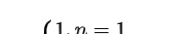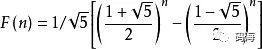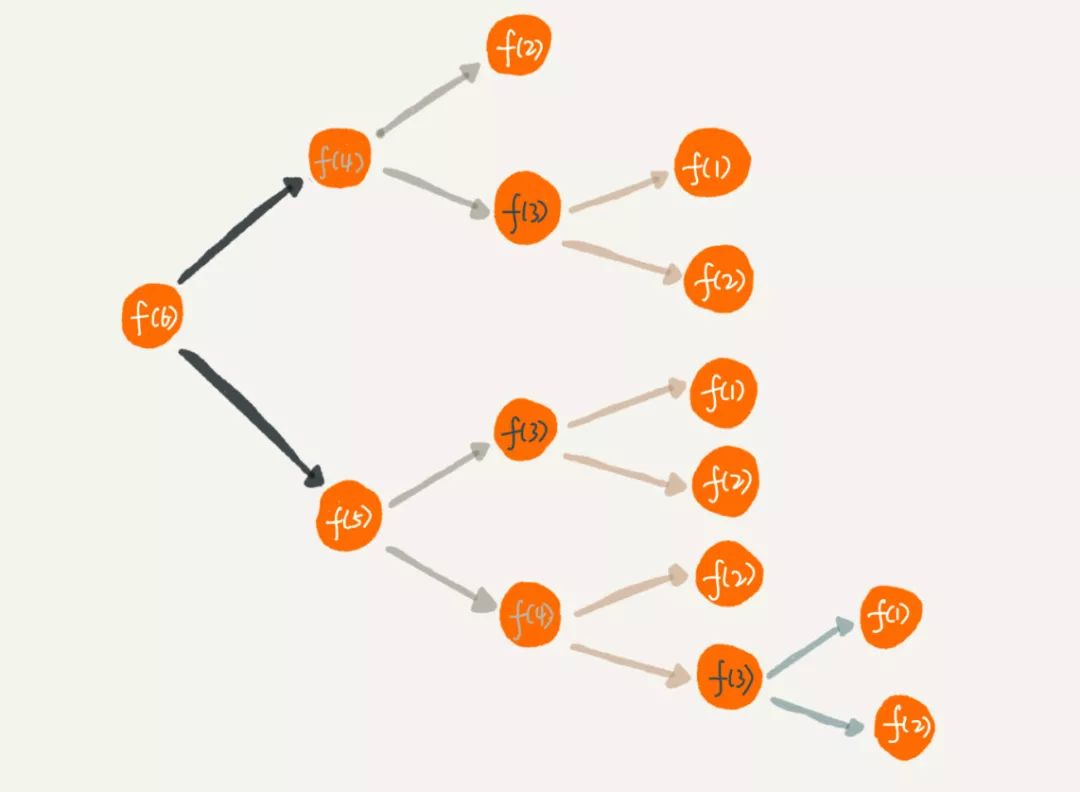5.优化

``````public int f(int n){
if (n == 1) return1;
if (n == 2) return2;
// map 即保存中间态的键值对， key 为 n，value 即 f(n)
if (map.get(n)) {
return map.get(n)
}
return f(n-1) + f(n-2)
}``````

6.使用循环迭代来改造算法 我们在分析问题与子问题关系（f(n) = f(n-1) + f(n-2)）的时候用的是自顶向下的分析方式,但其实我们在解 f(n) 的时候可以用自下而上的方式来解决，通过观察我们可以发现以下规律

``````f(1) = 1
f(2) = 2
f(3) = f(1) + f(2) = 3
f(4) = f(3) + f(2) = 5
....
f(n) = f(n-1) + f(n-2)``````

``````public int f(int n){
if (n == 1) return1;
if (n == 2) return2;

int result = 0;
int pre = 1;
int next = 2;

for (int i = 3; i < n + 1; i ++) {
result = pre + next;
pre = next;
next = result;
}
return result;
}``````

#### 初级题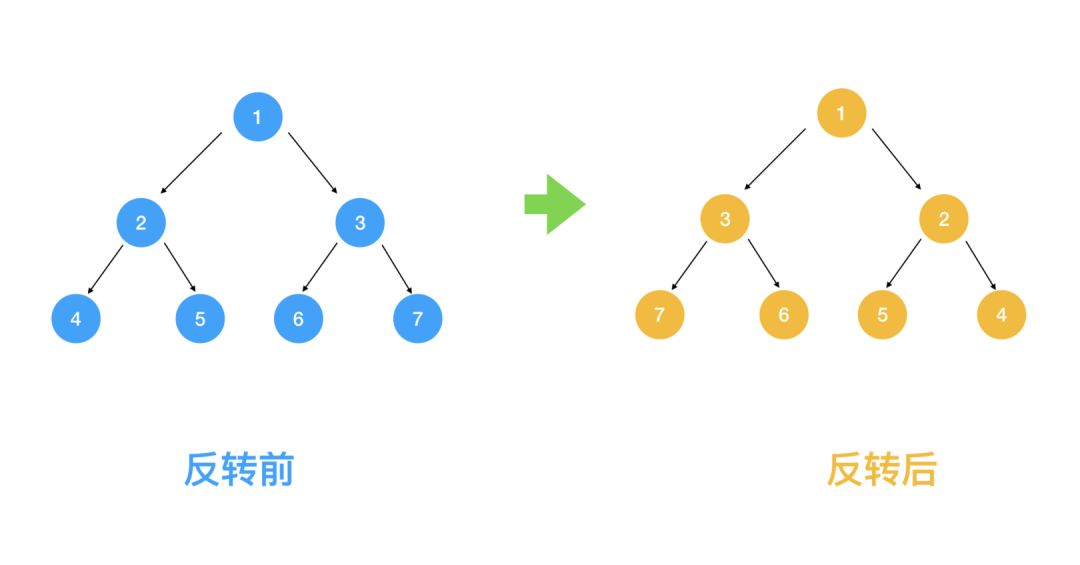1.定义一个函数，这个函数代表了翻转以 root 为根节点的二叉树

``````publicstaticclass TreeNode {
int val;
TreeNode left;
TreeNode right;
TreeNode(int x) { val = x; }
}

public TreeNode invertTree(TreeNode root){
}``````

2.查找问题与子问题的关系,得出递推公式 我们之前说了，解题要采用自上而下的思考方式，那我们取前面的1， 2，3 结点来看，对于根节点 1 来说，假设 2, 3 结点下的节点都已经翻转，那么只要翻转 2， 3 节点即满足需求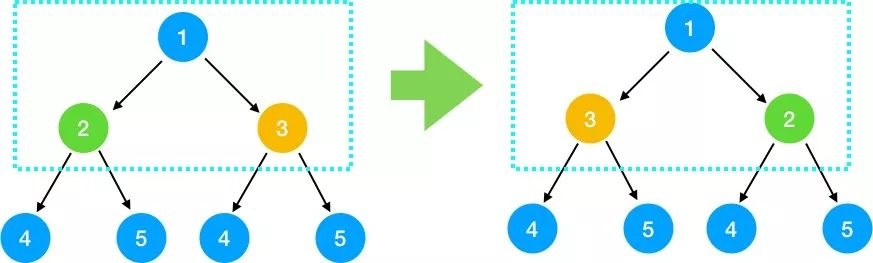invert(root) = invert(root->left) + invert(root->right)

3.将第二步的递推公式用代码表示出来补充到步骤 1 定义的函数中

``````public TreeNode invertTree(TreeNode root){
// 叶子结果不能翻转
if (root == null) {
returnnull;
}
// 翻转左节点下的左右节点
TreeNode left = invertTree(root.left);
// 翻转右节点下的左右节点
TreeNode right = invertTree(root.right);

// 左右节点下的二叉树翻转好后，翻转根节点的左右节点
root.right = left;
root.left = right;
return root;
}``````

4.时间复杂度分析 由于我们会对每一个节点都去做翻转，所以时间复杂度是 O(n)，那么空间复杂度呢，这道题的空间复杂度非常有意思，我们一起来看下，由于每次调用 invertTree 函数都相当于一次压栈操作， 那最多压了几次栈呢， 仔细看上面函数的下一段代码

``    TreeNode left = invertTree(root.left);``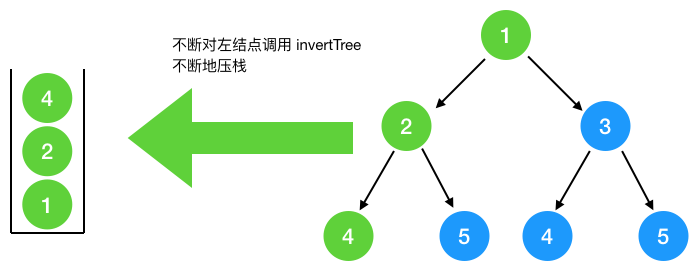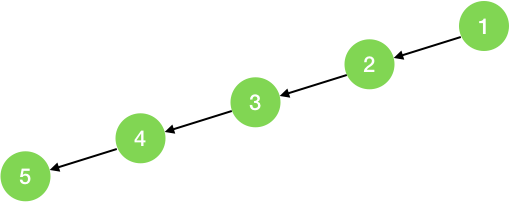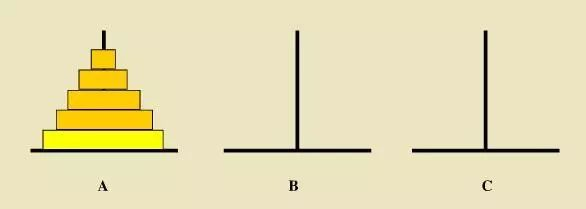1.定义问题的递归函数，明确函数的功能,我们定义这个函数的功能为：把 A 上面的 n 个圆盘经由 B 移到 C

``````// 将 n 个圆盘从 a 经由 b 移动到 c 上
public void hanoid(int n, char a, char b, char c){
}``````

2.查找问题与子问题的关系 首先我们看如果 A 柱子上只有两块圆盘该怎么移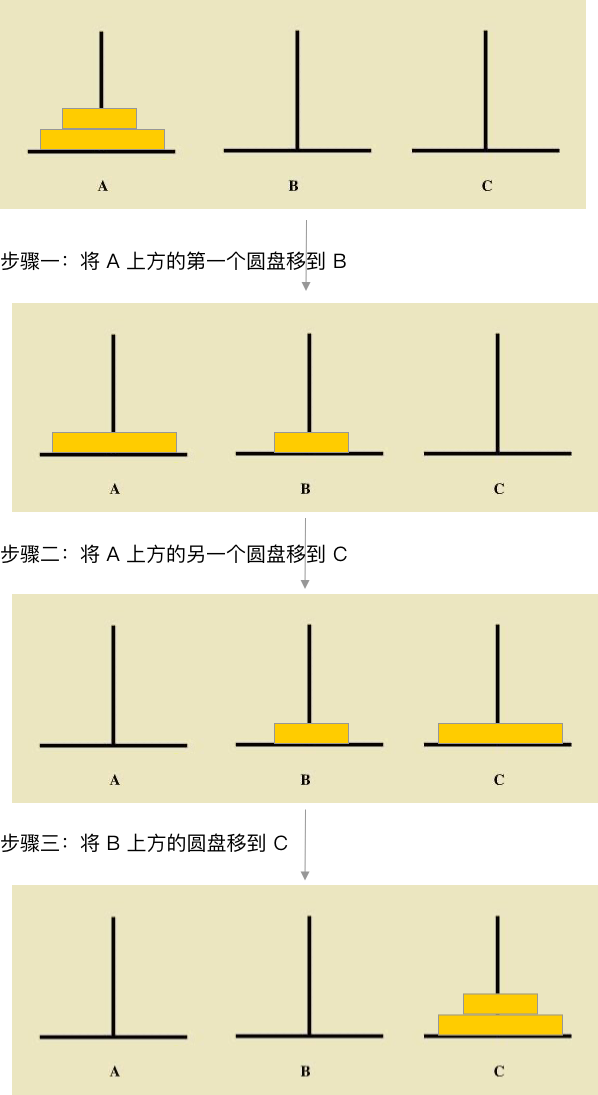move(n from A to C) = move(n-1 from A to B) + move(A to C) + move(n-1 from B to C`)

3.根据以上的递归伪代码补充函数的功能

``````// 将 n 个圆盘从 a 经由 b 移动到 c 上
public void hanoid(int n, char a, char b, char c){
if (n <= 0) {
return;
}
// 将上面的  n-1 个圆盘经由 C 移到 B
hanoid(n-1, a, c, b);
// 此时将 A 底下的那块最大的圆盘移到 C
move(a, c);
// 再将 B 上的 n-1 个圆盘经由A移到 C上
hanoid(n-1, b, a, c);
}

public void move(char a, char b){
printf("%c->%c\n", a, b);
}``````

4.时间复杂度分析 从第三步补充好的函数中我们可以推断出

f(n) = f(n-1) + 1 + f(n-1) = 2f(n-1) + 1 = 2(2f(n-2) + 1) + 1 = 2 * 2 * f(n-2) + 2 + 1 = 22 * f(n-3) + 2 + 1 = 22 * f(n-3) + 2 + 1 =  22 * (2f(n-4) + 1) = 23 * f(n-4) + 22  + 1 = ....        // 不断地展开 = 2n-1 + 2n-2 + ....+ 1

#### 进阶题

1.定义问题的递归函数，明确函数的功能 我们定义以下函数为 n 个小时后的细胞数

``````public int allCells(int n){
}``````

2.接下来寻找问题与子问题间的关系（即递推公式） 首先我们看一下一个细胞出生到死亡后经历的所有细胞分裂过程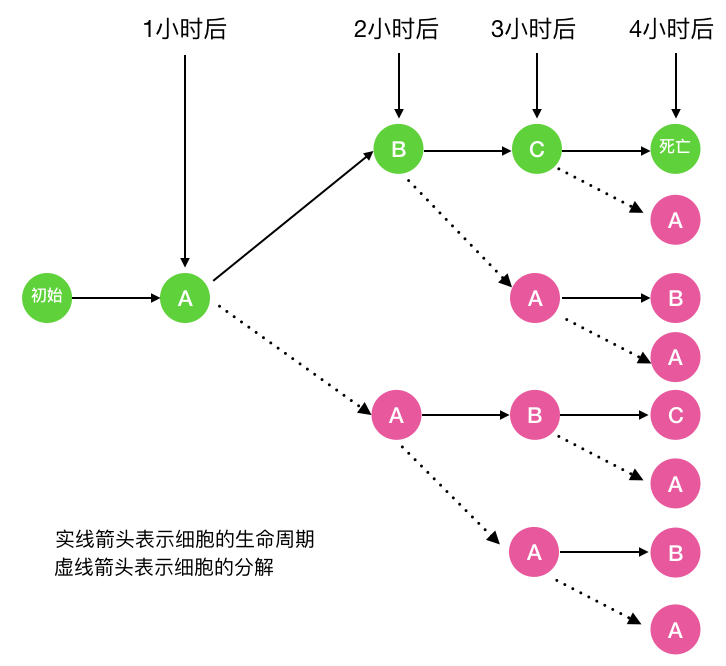fb(n) 呢,看下图可知 fb(n)  = fa(n-1)。当 n = 1 时  fb(n) = 0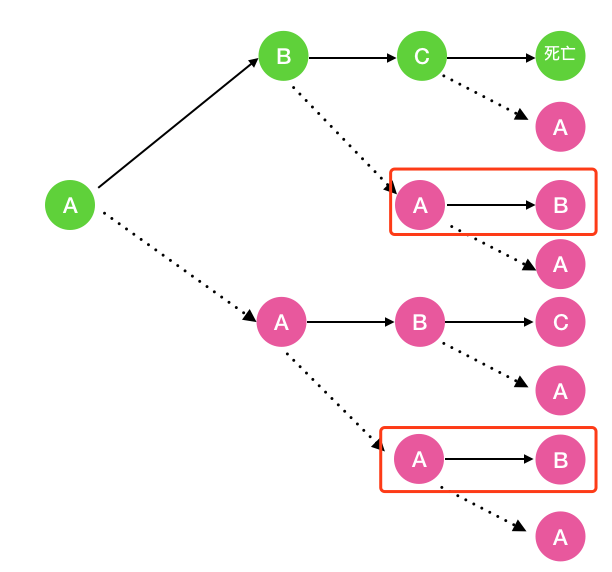fc(n) 呢,看下图可知  fc(n)  = fb(n-1)。当 n = 1,2 时 fc(n) = 0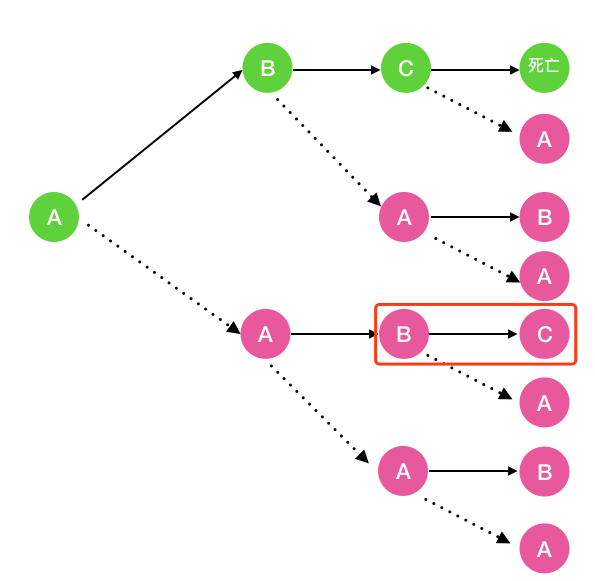f(n) =  fa(n)  + fb(n)  + fc(n)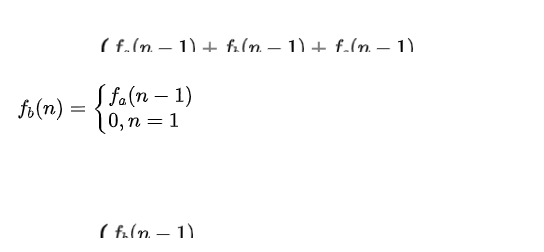3.根据以上递归公式我们补充一下函数的功能

``````public int allCells(int n){
return aCell(n) + bCell(n) + cCell(n);
}

/**
* 第 n 小时 a 状态的细胞数
*/
public int aCell(int n){
if(n==1){
return1;
}else{
return aCell(n-1)+bCell(n-1)+cCell(n-1);
}
}

/**
* 第 n 小时 b 状态的细胞数
*/
public int bCell(int n){
if(n==1){
return0;
}else{
return aCell(n-1);
}
}

/**
* 第 n 小时 c 状态的细胞数
*/
public int cCell(int n){
if(n==1 || n==2){
return0;
}else{
return bCell(n-1);
}
}``````

4.求时间复杂度 由第二步的递推公式我们知道 f(n) = 2aCell(n-1) + 2aCell(n-2) + aCell(n-3)

### 总结帖子
视频
声望
粉丝
社区精华内容